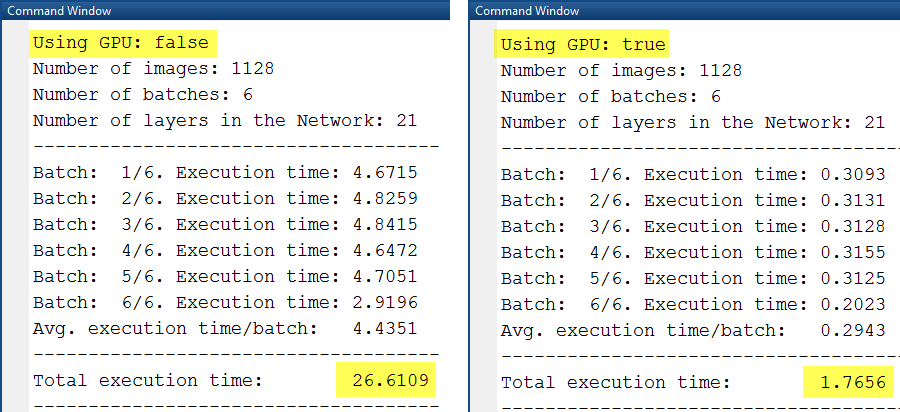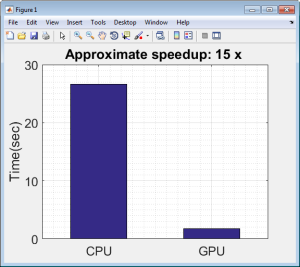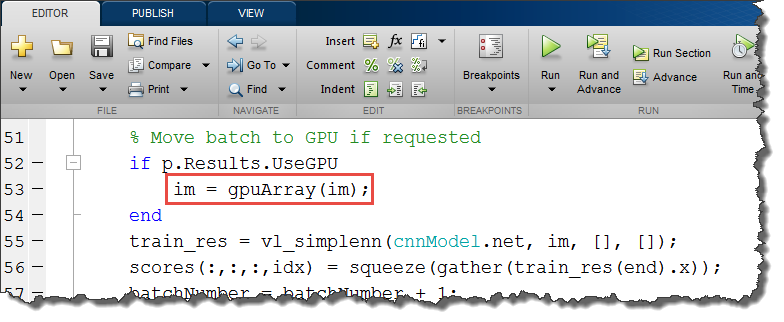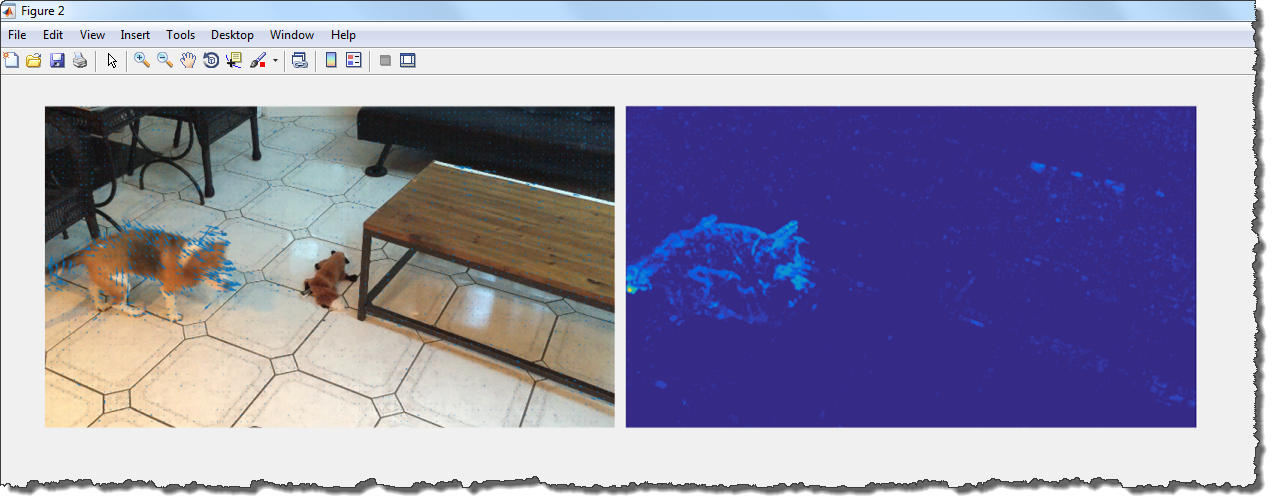# Deep Learning for Computer Vision with MATLAB and cuDNNDeep learning is becoming ubiquitous. With recent advancements in deep learning algorithms and GPU technology, we are able to solve problems once considered impossible in fields such as computer vision, natural language processing, and robotics.

Deep learning uses deep neural networks which have been around for a few decades; what’s changed in recent years is the availability of large labeled datasets and powerful GPUs. Neural networks are inherently parallel algorithms and GPUs with thousands of cores can take advantage of this parallelism to dramatically reduce computation time needed for training deep learning networks. In this post, I will discuss how you can use MATLAB to develop an object recognition system using deep convolutional neural networks and GPUs.

## Why Deep Learning for Computer Vision?

Machine learning techniques use data (images, signals, text) to train a machine (or model) to perform a task such as image classification, object detection, or language translation. Classical machine learning techniques are still being used to solve challenging image classification problems. However, they don’t work well when applied directly to images, because they ignore the structure and compositional nature of images. Until recently, state-of-the-art techniques made use of feature extraction algorithms that extract interesting parts of an image as compact low-dimensional feature vectors. These were then used along with traditional machine learning algorithms.

Enter Deep learning. Deep convolutional neural networks (CNNs), a specific type of deep learning algorithm, address the gaps in traditional machine learning techniques, changing the way we solve these problems. CNNs not only perform classification, but they can also learn to extract features directly from raw images, eliminating the need for manual feature extraction. For computer vision applications you often need more than just image classification; you need state-of-the-art computer vision techniques for object detection, a bit of domain expertise, and the know-how to set up and use GPUs efficiently. Through the rest of this post, I will use an object recognition example to illustrate how easy it is to use MATLAB for deep learning, even if you don’t have extensive knowledge of computer vision or GPU programming.

## Example: Object Detection and Recognition

The goal in this example is to detect a pet in a video and correctly label the pet as a cat or a dog. To run this example, you will need MATLAB®, Parallel Computing Toolbox™, Computer Vision System Toolbox™ and Statistics and Machine Learning Toolbox™. If you don’t have these tools, request a trial at www.mathworks.com/trial. For this problem I used an NVIDIA Tesla K40 GPU; you can run it on any MATLAB compatible CUDA-enabled NVIDIA GPU.

Our approach involves two steps:

1. Object Detection: “Where is the pet in the video?”
2. Object Recognition: “Now that I know where it is, is it a cat or a dog?”

Figure 1 shows what the final result looks like.

### Using a Pretrained CNN Classifier

The first step is to train a classifier that can classify images of cats and dogs. I could either:

1. Collect a massive amount of cropped, resized and labeled images of cats and dogs in a reasonable amount of time (good luck!), or
2. Use a model that has already been trained on a variety of common objects and adapt it for my problem.

For this example, I’m going to go with option (2) which is common in practice. To do that I’m going to first start with a pretrained CNN classifier that has been trained on the ImageNet dataset.

I will be using MatConvNet, a CNN package for MATLAB that uses the NVIDIA cuDNN library for accelerated training and prediction. [To learn more about cuDNN, see this Parallel Forall post.] Download and install instructions for MatConvNet are available on its home page. Once I’ve installed MatConvNet on my computer, I can use the following MATLAB code to download and make predictions using the pretrained CNN classifier. Note: I also use the `cnnPredict()` helper function, which I’ve made available on Github.

```%% Download and predict using a pretrained ImageNet model

% Setup MatConvNet
run(fullfile('matconvnet-1.0-beta15','matlab','vl_setupnn.m'));

urlwrite('http://www.vlfeat.org/matconvnet/models/imagenet-vgg-f.mat', 'imagenet-vgg-f.mat');

% Load and display an example image
imshow('dog_example.png');

% Predict label using ImageNet trained vgg-f CNN model
label = cnnPredict(cnnModel,img);
title(label,'FontSize',20)```

The pretrained CNN classifier works great out of the box at object classification. The CNN model is able to tell me that there is a beagle in the example image (Figure 2). While this is certainly a great starting point, our problem is a little different. I want to be able to (1) put a box around where the pet is (object detection) and then (2) label it accurately as a dog or a cat (classification). Let’s start by building a dog vs cat classifier from the pretrained CNN model.

### Training a Dog vs. Cat Classifier

The objective is simple. I want to solve a simple classification task: given an image I’d like to train a classifier that can accurately tell me if it’s an image of a dog or a cat. I can do that easily with this pretrained classifier and a few dog and cat images.

To get a small collection of labeled images for this project, I went around my office asking colleagues to send me pictures of their pets. I segregated the images and put them into separate ‘cat’ and ‘dog’ folders under a parent called ‘pet_images’. The advantage of using this folder structure is that the `imageSet` function can automatically manage image locations and labels. I loaded them all into MATLAB using the following code.

```%% Load images from folder
% Use imageSet to load images stored in pet_images folder
imset = imageSet('pet_images','recursive');

% Preallocate arrays with fixed size for prediction
imageSize = cnnModel.net.normalization.imageSize;
trainingImages = zeros([imageSize sum([imset(:).Count])],'single');

% Load and resize images for prediction
for ii = 1:numel(imset)
for jj = 1:imset(ii).Count
end
end

% Get the image labels
trainingLabels = getImageLabels(imset);
summary(trainingLabels) % Display class label distribution```

### Feature Extraction using a CNN

What I’d like to do next is use this new dataset along with the pretrained ImageNet to extract features. As I mentioned earlier, CNNs can learn to extract generic features from images. These features can be used to train a new classifier to solve a different problem, like classifying cats and dogs in our problem.

CNN algorithms are compute-intensive and can be slow to run. Since they are inherently parallel algorithms, I can use GPUs to speed up the computation. Here is the code that performs the feature extraction using the pretrained model, and a comparison of multithreaded CPU (Intel Core i7-3770 CPU) and GPU (NVIDIA Tesla K40 GPU) implementations.

```%% Extract features using pretrained CNN

% Depending on how much memory you have on your GPU you may use a larger
% batch size. I have 400 images, so I choose 200 as my batch size
cnnModel.info.opts.batchSize = 200;

% Make prediction on a CPU
[~, cnnFeatures, timeCPU] = cnnPredict(cnnModel,trainingImages,'UseGPU',false);
% Make prediction on a GPU
[~, cnnFeatures, timeGPU] = cnnPredict(cnnModel,trainingImages,'UseGPU',true);

% Compare the performance increase
bar([sum(timeCPU),sum(timeGPU)],0.5)
title(sprintf('Approximate speedup: %2.00f x ',sum(timeCPU)/sum(timeGPU)))
set(gca,'XTickLabel',{'CPU','GPU'},'FontSize',18)
ylabel('Time(sec)'), grid on, grid minor```Figure 3: Comparision of execution times for feature extraction using a CPU (left) and NVIDIA Tesla K40 GPU (right).Figure 4: The CPU and GPU time required to extract features from 1128 images.

As you can see the performance boost you get from using a GPU is significant, about 15x for this feature extraction problem.

The function `cnnPredict` is a wrapper around MatConvNet’s `vl_simplenn` predict function. The highlighted line of code in Figure 5 is the only modification you need to make to run the prediction on a GPU. Functions like `gpuArray` in the Parallel Computing Toolbox make it easy to prototype your algorithms using a CPU and quickly switch to GPUs with minimal code changes.Figure 5: The `gpuArray` and `gather` functions allow you to transfer data from the MATLAB workspace to the GPU and back.

### Train a Classifier Using CNN Features

With the features I extracted in the previous step, I’m now ready to train a “shallow” classifier. To train and compare multiple models interactively, I can use the Classification Learner app in the Statistics and Machine Learning Toolbox. Note: for an introduction to machine learning and classification workflows in MATLAB, check out this Machine Learning Made Easy webinar.

Next, I will directly train an SVM classifier using the extracted features by calling the `fitcsvm` function using `cnnFeatures` as the input or predictors and `trainingLabels` as the output or response values. I will also cross-validate the classifier to test its validation accuracy. The validation accuracy is an unbiased estimate of how the classifier would perform in practice on unseen data.

```%% Train a classifier using extracted features

% Here I train a linear support vector machine (SVM) classifier.
svmmdl = fitcsvm(cnnFeatures,trainingLabels);

% Perform crossvalidation and check accuracy
cvmdl = crossval(svmmdl,'KFold',10);
fprintf('kFold CV accuracy: %2.2f\n',1-cvmdl.kfoldLoss)```

`svmmdl` is my classifier that I can now use to classify an image as a cat or a dog.

### Object Detection

Most images and videos frames have a lot going on in them. In addition to a dog, there may be a tree or a raccoon chasing the dog. Even with a great image classifier, like the one I built in the previous step, it will only work well if I can locate the object of interest in an image (dog or cat), crop the object and then feed it to a classifier. The step of locating the object is called object detection.

For object detection, I will use a technique called Optical Flow that uses the motion of pixels in a video from frame to frame. Figure 6 shows a single frame of video with the motion vectors overlaid.Figure 6: A single frame of video with motion vectors overlaid (left) and magnitude of the motion vectors (right).

The next step in the detection process is to separate out pixels that are moving, and then use the Image Region Analyzer app to analyze the connected components in the binary image to filter out the noisy pixels caused by the camera motion. The output of the app is a MATLAB function (I’m going to call it findPet) that can locate where the pet is in the field of view.

## Tying the Workflow Together

I now have all the pieces I need to build a pet detection and recognition system.

To quickly recap, I can:

• Detect the location of the pet in new images;
• Crop the pet from the image and extract features using a pretrained CNN;
• Classify the features using an SVM classifier.

### Pet Detection and Recognition

Tying all these pieces together, the following code shows my complete MATLAB pet detection and recognition system.

```%% Tying the workflow together
vw = VideoWriter('test.avi','Motion JPEG AVI');
opticFlow = opticalFlowFarneback;
open(vw);

while hasFrame(vr)
% Count frames

% Step 2. Detect ROI
vFrame = imresize(videoFrame,0.25); % Get video frame
frameGray = rgb2gray(vFrame); % Convert to gray for detection
bboxes = findPet(frameGray,opticFlow); % Find bounding boxes
if ~isempty(bboxes)
img = zeros([imageSize size(bboxes,1)]);
for ii = 1:size(bboxes,1)
img(:,:,:,ii) = imresize(imcrop(videoFrame,bboxes(ii,:)),imageSize(1:2));
end

% Step 3. Recognize object
% (a) Extract features using a CNN
[~, scores] = cnnPredict(cnnModel,img,'UseGPU',true,'display',false);

% (b) Predict using the trained SVM Classifier
label = predict(svmmdl,scores);

% Step 4. Annotate object
videoFrame = insertObjectAnnotation(videoFrame,'Rectangle',bboxes,cellstr(label),'FontSize',40);
end

% Step 5. Write video to file
writeVideo(vw,videoFrame);